Ex 4.1

Chapter 4 Class 11 Mathematical Induction
Serial order wise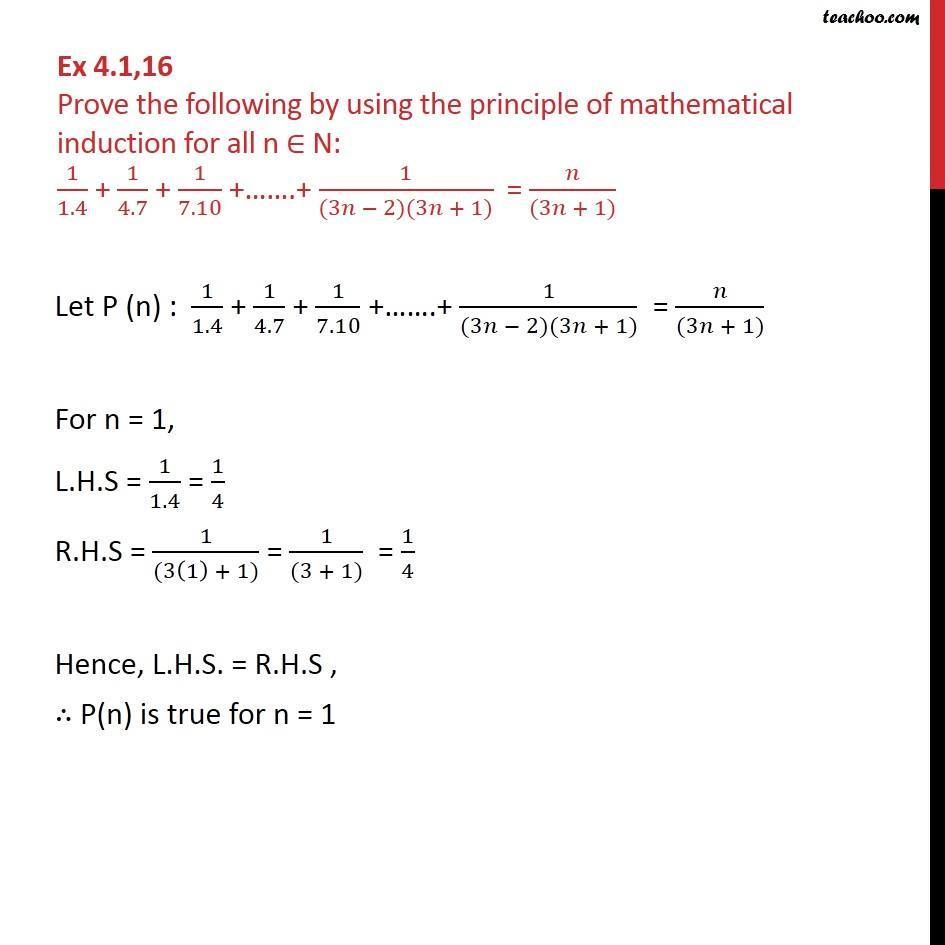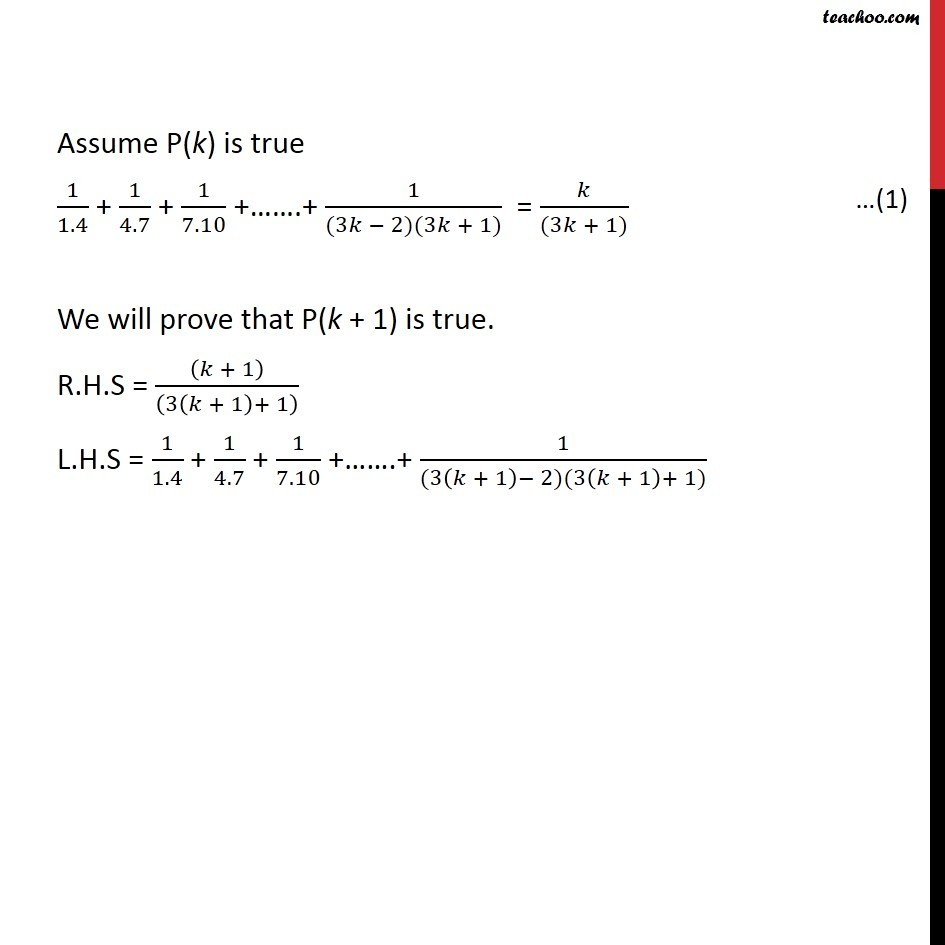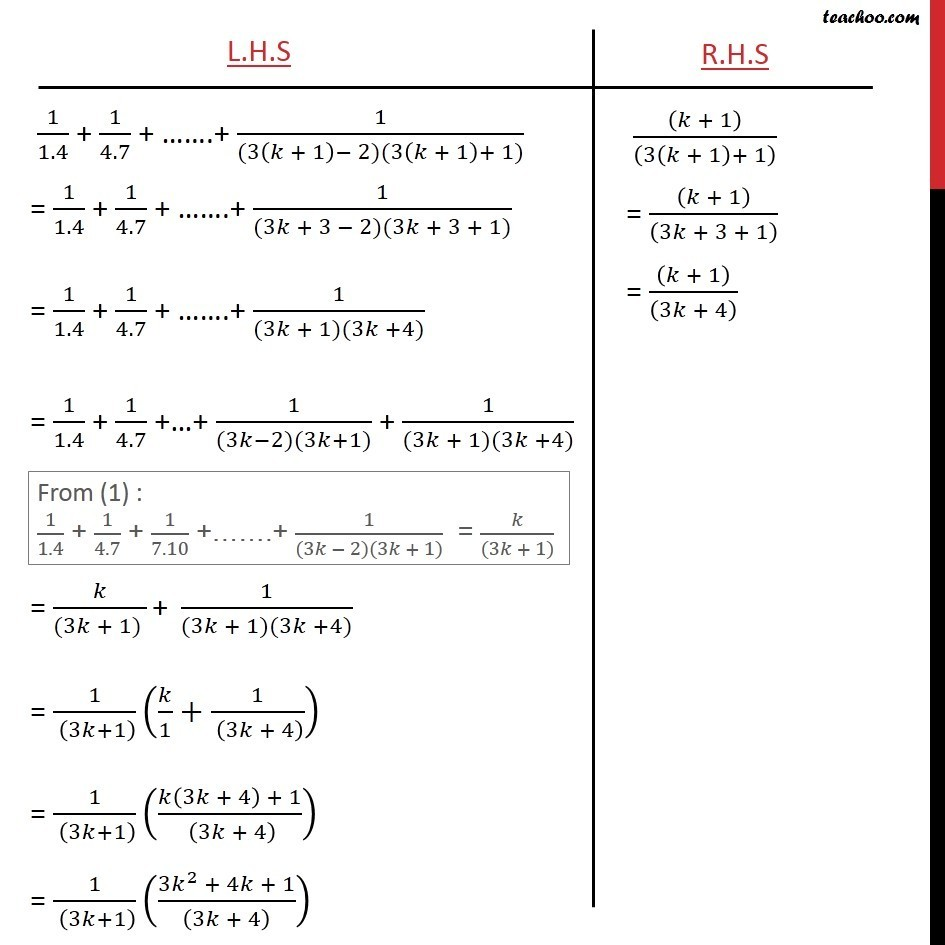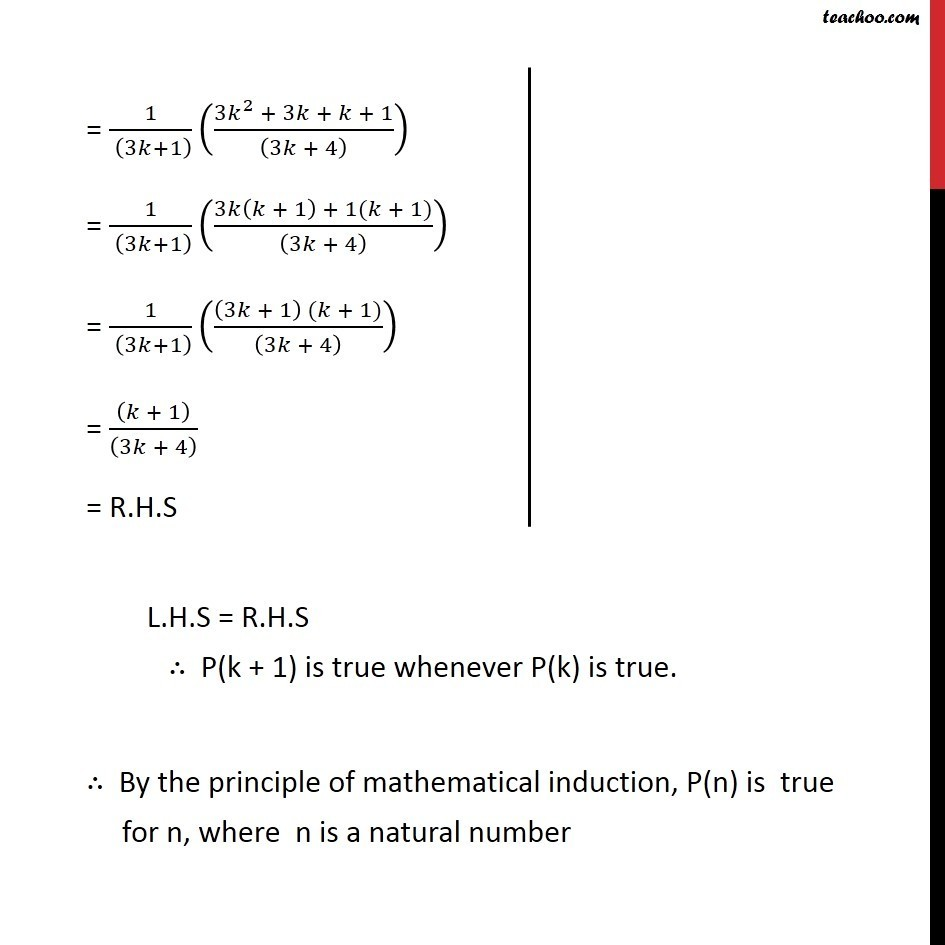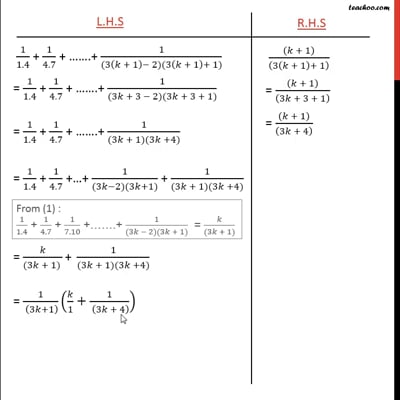This video is only available for Teachoo black users

Solve all your doubts with Teachoo Black (new monthly pack available now!)

### Transcript

Ex 4.1,16 Prove the following by using the principle of mathematical induction for all n ∈ N: 1/1.4 + 1/4.7 + 1/7.10 +…….+ 1/((3𝑛 − 2)(3𝑛 + 1)) = 𝑛/((3𝑛 + 1)) Let P (n) : 1/1.4 + 1/4.7 + 1/7.10 +…….+ 1/((3𝑛 − 2)(3𝑛 + 1)) = 𝑛/((3𝑛 + 1)) For n = 1, L.H.S = 1/1.4 = 1/4 R.H.S = 1/((3(1) + 1)) = 1/((3 + 1)) = 1/4 Hence, L.H.S. = R.H.S , ∴ P(n) is true for n = 1 Assume P(k) is true 1/1.4 + 1/4.7 + 1/7.10 +…….+ 1/((3𝑘 − 2)(3𝑘 + 1)) = 𝑘/((3𝑘 + 1)) We will prove that P(k + 1) is true. R.H.S = ((𝑘 + 1))/((3(𝑘 + 1)+ 1) ) L.H.S = 1/1.4 + 1/4.7 + 1/7.10 +…….+ 1/((3(𝑘 + 1)− 2)(3(𝑘 + 1)+ 1))